# NormalNoiseProperties

Demonstrates the computation of properties for normally distributed noise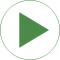# Information

This information is part of the Modelica Standard Library maintained by the Modelica Association.

This example demonstrates statistical properties of the Blocks.Noise.NormalNoise block using a normal random number distribution with mu=3, sigma=1. From the generated noise the mean and the variance is computed with blocks of package Blocks.Math. Simulation results are shown in the next diagram: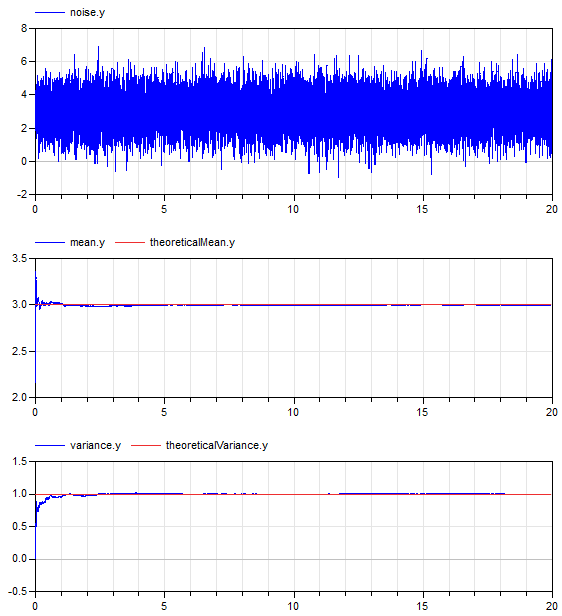The mean value of a normal noise with mu=3 is 3 and the variance of normal noise is sigma^2, so 1. The simulation results above show good agreement (after a short initial phase). This demonstrates that the random number generator and the mapping to a normal distribution have good statistical properties.

# Parameters (5)

mu Value: 3 Type: Real Description: Mean value for normal distribution Value: 1 Type: Real Description: Standard deviation for normal distribution Value: mu Type: Real Description: Theoretical mean value of normal distribution Value: sigma ^ 2 Type: Real Description: Theoretical variance of uniform distribution Value: sigma Type: Real Description: Theoretical standard deviation of normal distribution

# Outputs (2)

meanError_y Default Value: meanError.y Type: Real Default Value: sigmaError.y Type: Real

# Components (11)

globalSeed noise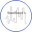Type: GlobalSeed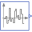Type: NormalNoise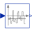Type: ContinuousMean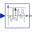Type: Variance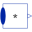Type: MultiProduct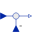Type: Feedback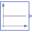Type: ConstantType: FeedbackType: Constant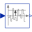Type: StandardDeviationType: Feedback